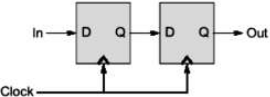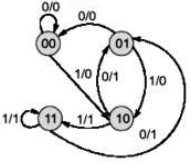# GATE Solved Paper 2017-19 - GATE 2018

The number of states in the state transition diagram of the circuit that have a transition back to the same state on some value of “in” is __________.

Note – Numerical Type question

• A

2• B

3• C

4• D

5• Option : A
• Explanation :State Table:  P.S Input FF input N.S Q1 Q0 x D1 = x D1 = Q1 Q1 Q0 Out = Q0 0 0 0 0 0 0 0 0 0 0 1 1 0 1 0 0 0 1 0 0 0 0 0 0 0 1 1 1 0 1 0 0 1 0 0 0 1 0 1 1 1 0 1 1 1 1 1 1 1 1 0 0 1 0 1 1 1 1 1 1 1 1 1 1
State Transition Diagram:self-loop states are 00 and 11.

• A

33• B

44• C

34• D

35• Option : C
• Explanation :
1 sec = 109 bits
(232 x 8)/109 = bytes
⇒ 34.35 sec

• A

75• B

70• C

80• D

85• Option : D
• Explanation :(86 - 80) + (86 - 72) + (134 - 72) + (134 - 16) + 62 + 118 + 14 = 200
100 → 20
200 → ?
(200/100) x 20 = 40
3 direction changes 3 x 15 = 45
40 + 45 = 85

• A

32• B

64• C

256• D

512• Option : A
• Explanation :
Given, size of instruction format is 2 byte (= 16 bits), therefore number of instruction encoding = 216
Also, total number of bits in integer operand = log2(16 integer registers) = 4
Total number of bits in floating point operand = log2(64 floating point registers) = 6
So, number of encoding consumed:

By type 1 instructions = 4×23×4 = 214
By type 2 instructions = 8×22×6 = 215
By type 3 instructions = 14×2(4+6) = 14336

Now, number of encoding left for type 4 instructions = 216 − (214 + 215 + 14336) = 2048
Therefore, total number of different instructions of type 4 instructions = 2048 /64 = 32 Please note that there is difference between number of different instructions and number of different encoding, a single instruction can have different encodings when the address part differs. So, answer is 32.

Choose a value of x that will maximize the number of minimum weight spanning trees (MWSTs) of G. The number of MWSTs of G for this value of x is ___________.

Note – Numerical Type question

• A

4• B

5• C

2• D

3• Option : A
• Explanation :
ü Edges with weights 1 and 3 will be selected first,
ü Now bottom edge with weight 4 will not be selected as will cause cycle on MST,
ü both corner vertices have two-two choices to select the vertices, so these corner edges with weights 4 and 5 will resultant 2*2 = 4 MSTs.
So, total number of MSTs are 2*2 = 4, which is answer.
Option (A) is correct.
Related Quiz.
GATE 2018